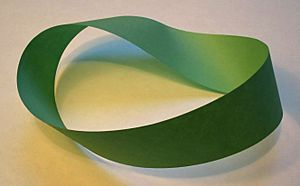# Topology facts for kids

Kids Encyclopedia Facts

Topology is the study of how spaces are organized, how the objects are structured in terms of position. It also studies how spaces are connected. It is divided into algebraic topology, differential topology and geometric topology.A Möbius strip, a surface with only one side and one edge; such shapes are an object of study in topology.

Topology has sometimes been called rubber-sheet geometry, because in topology of 2 dimensions, there is no difference between a circle and a square (a circle made out of a rubber band can be stretched into a square) but there is a difference between a circle and a figure eight (you cannot stretch a figure eight into a circle without tearing). The spaces studied in topology are called topological spaces. They vary from familiar manifolds to some very exotic constructions.

## Natural Origin

In many problems, we often divide a large space into smaller areas, for instance, a house is divided into rooms, a nation into states, a type of quantity into numbers, etc. Each of these smaller areas (room, state, number) is next to other small areas (other rooms/states/numbers), and the places where the areas meet are connections. If we write down on paper a list of spaces, and the connections between them, we have written down a description of a space -- a topological space. All topological spaces have the same properties (connections, etc.) and are made of the same structure (a list of smaller areas). This makes it easier to study how spaces behave in general, and to use general algorithms. For instance, to program a robot to navigate a house, we simply give it a list of rooms, the connections between each room (doors, etc.), and an algorithm that can determine the sequence of rooms to travel through to reach any other desired room.

We can go further by creating subdivisions of subdivisions of space. For instance, a nation divided into states, divided into counties, divided into city boundaries, etc. All this kind of information can be described using topology.

## Images for kidsTopology Facts for Kids. Kiddle Encyclopedia.